Examples

Chapter 12 Class 9 Herons Formula
Serial order wise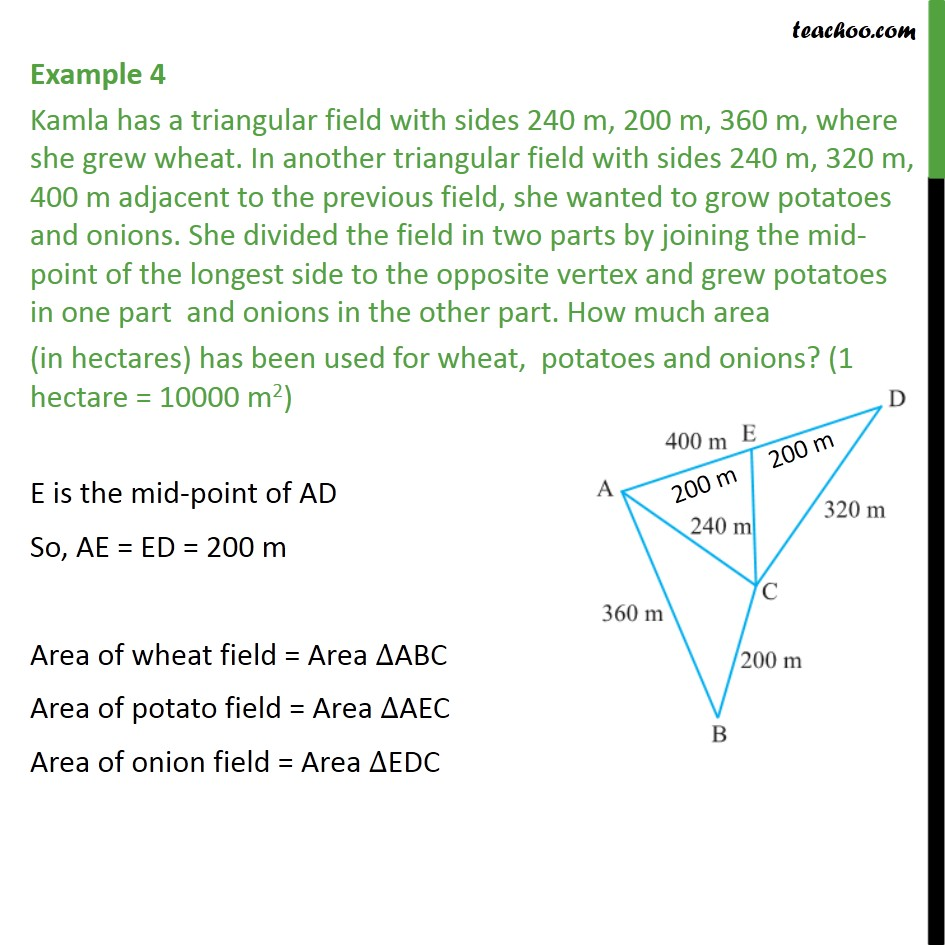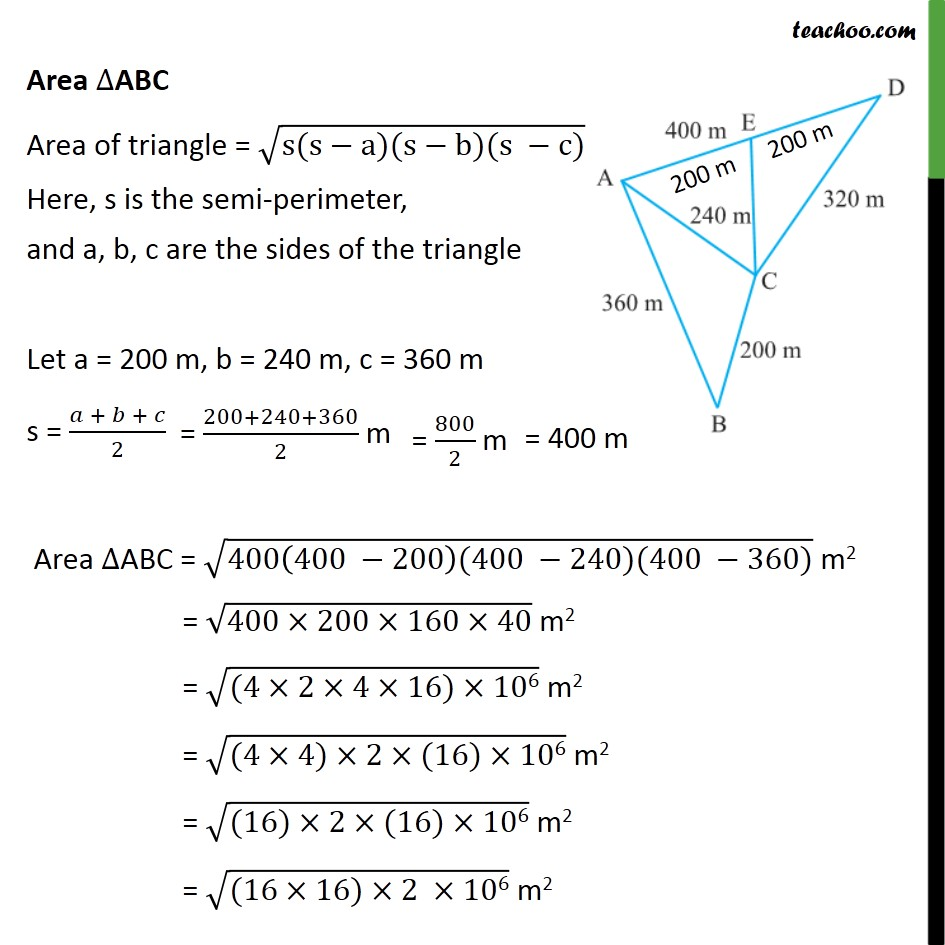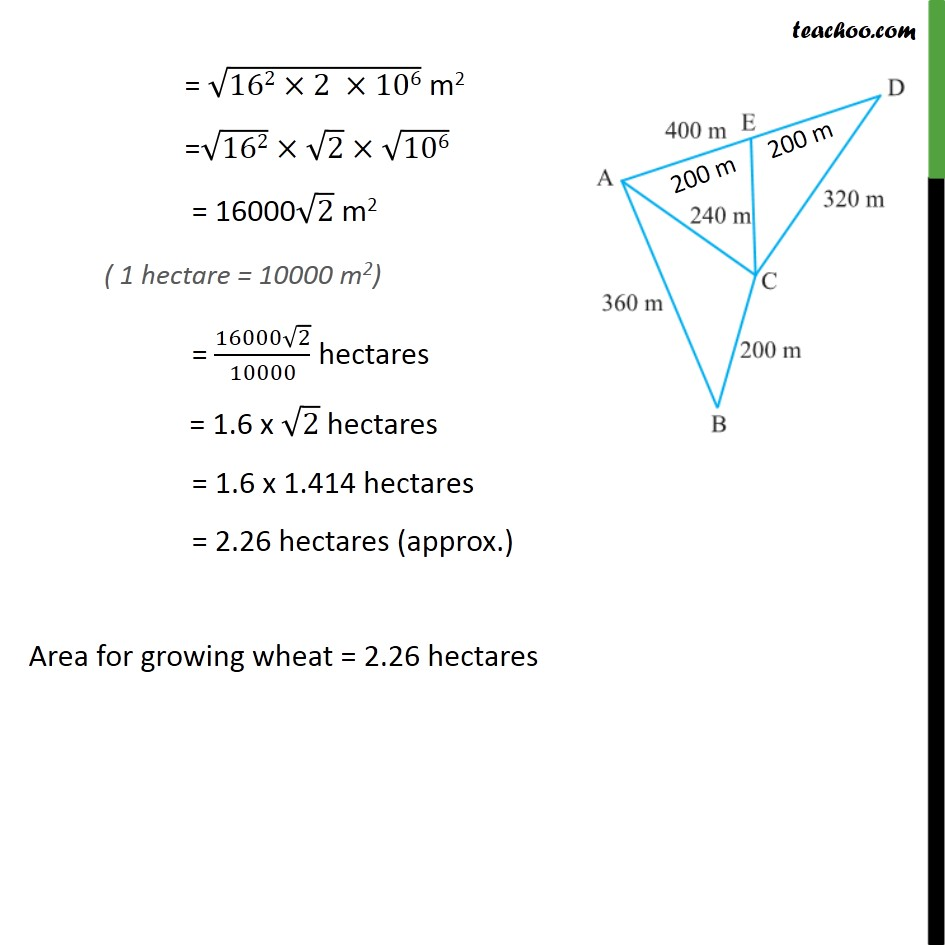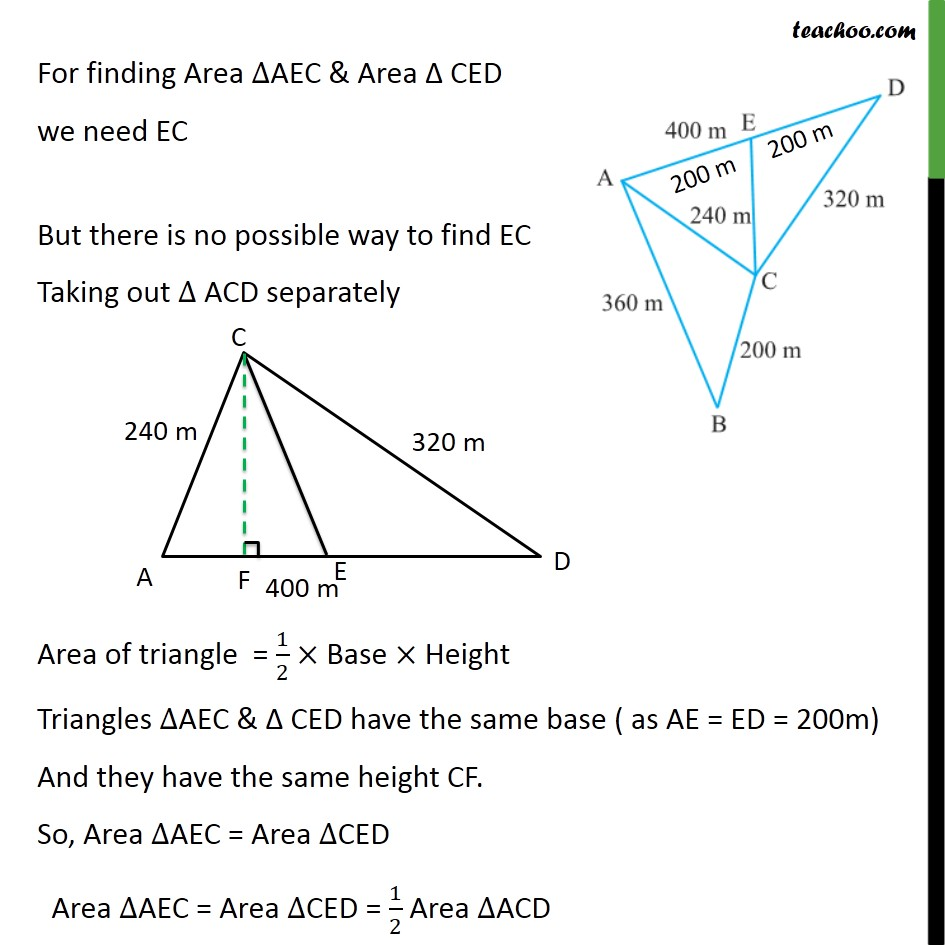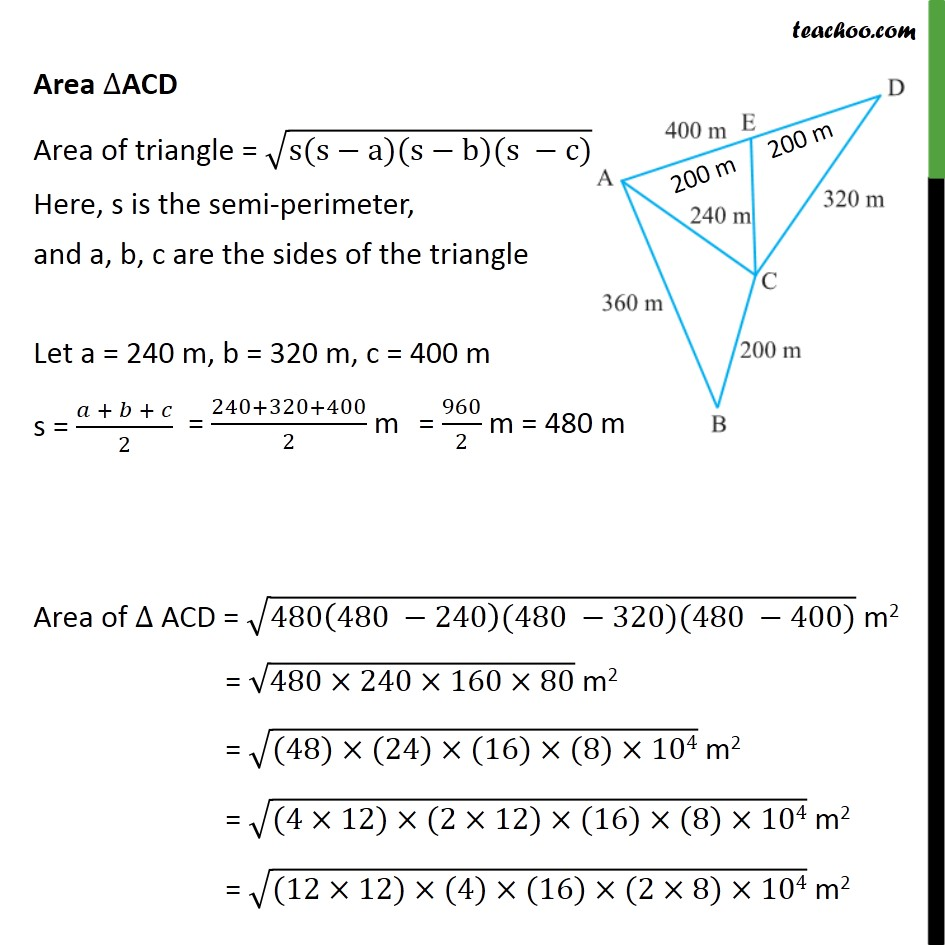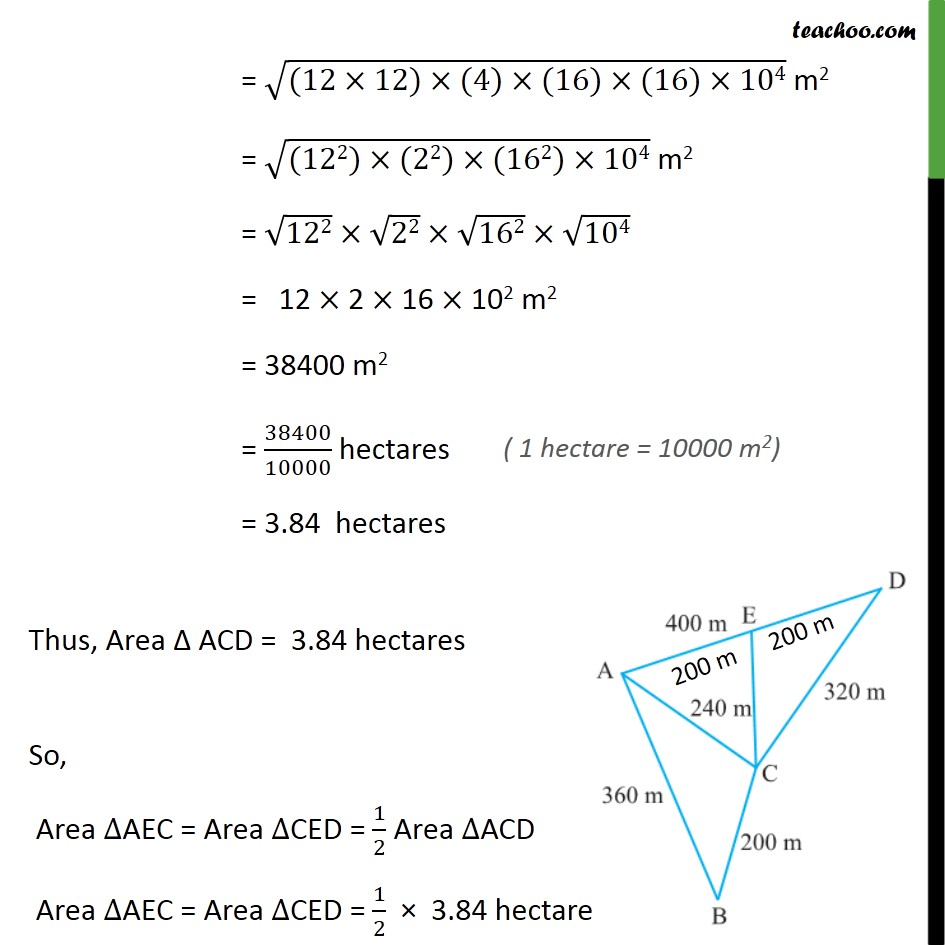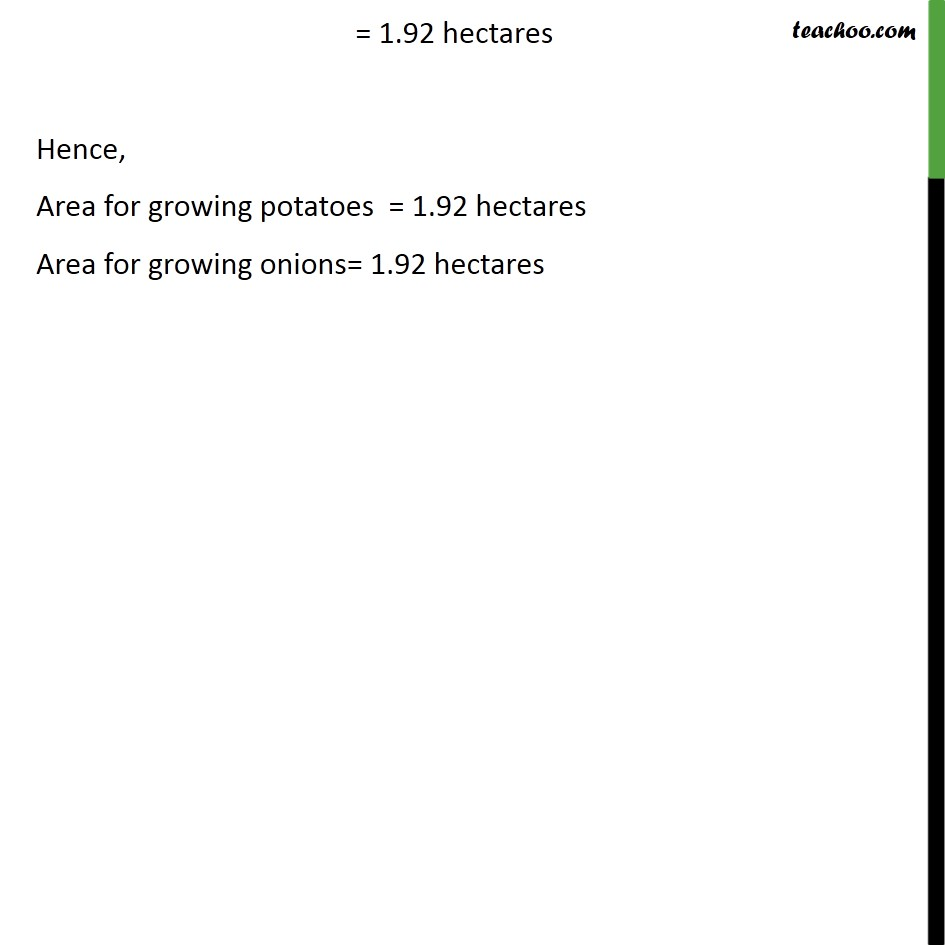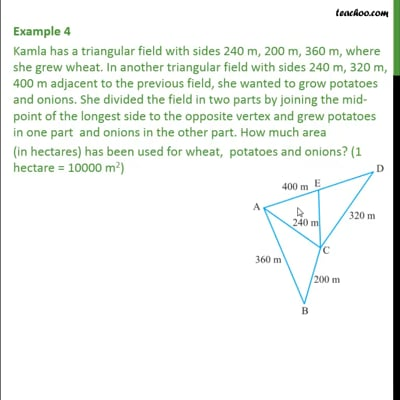This video is only available for Teachoo black users

Solve all your doubts with Teachoo Black (new monthly pack available now!)

### Transcript

Example 4 Kamla has a triangular field with sides 240 m, 200 m, 360 m, where she grew wheat. In another triangular field with sides 240 m, 320 m, 400 m adjacent to the previous field, she wanted to grow potatoes and onions. She divided the field in two parts by joining the mid-point of the longest side to the opposite vertex and grew potatoes in one part and onions in the other part. How much area (in hectares) has been used for wheat, potatoes and onions? (1 hectare = 10000 m2) E is the mid-point of AD So, AE = ED = 200 m Area of wheat field = Area ABC Area of potato field = Area AEC Area of onion field = Area EDC Area ABC Area of triangle = (s(s a)(s b)(s c)) Here, s is the semi-perimeter, and a, b, c are the sides of the triangle Let a = 200 m, b = 240 m, c = 360 m s = ( + + )/2 Area ABC = (400(400 200)(400 240)(400 360)) m2 = (400 200 160 40) m2 = ((4 2 4 16) 106) m2 = ((4 4) 2 (16) 106) m2 = ((16) 2 (16) 106) m2 = ((16 16) 2 106) m2 = (162 2 106) m2 = 162 2 106 = 16000 2 m2 = (16000 2)/10000 hectares = 1.6 x 2 hectares = 1.6 x 1.414 hectares = 2.26 hectares (approx.) Area for growing wheat = 2.26 hectares For finding Area AEC & Area CED we need EC But there is no possible way to find EC Taking out ACD separately Area of triangle = 1/2 Base Height Triangles AEC & CED have the same base ( as AE = ED = 200m) And they have the same height CF. So, Area AEC = Area CED Area AEC = Area CED = 1/2 Area ACD Area ACD Area of triangle = (s(s a)(s b)(s c)) Here, s is the semi-perimeter, and a, b, c are the sides of the triangle Let a = 240 m, b = 320 m, c = 400 m s = ( + + )/2 Area of ACD = (480(480 240)(480 320)(480 400)) m2 = (480 240 160 80) m2 = ((48) (24) (16) (8) 104) m2 = ((4 12) (2 12) (16) (8) 104) m2 = ((12 12) (4) (16) (2 8) 104) m2 = ((12 12) (4) (16) (16) 104) m2 = ((122) (22) (162) 104) m2 = 122 22 162 104 = 12 2 16 102 m2 = 38400 m2 = 38400/10000 hectares = 3.84 hectares Thus, Area ACD = 3.84 hectares So, Area AEC = Area CED = 1/2 Area ACD Area AEC = Area CED = 1/2 3.84 hectares = 1.92 hectares Hence, Area for growing potatoes = 1.92 hectares Area for growing onions= 1.92 hectares